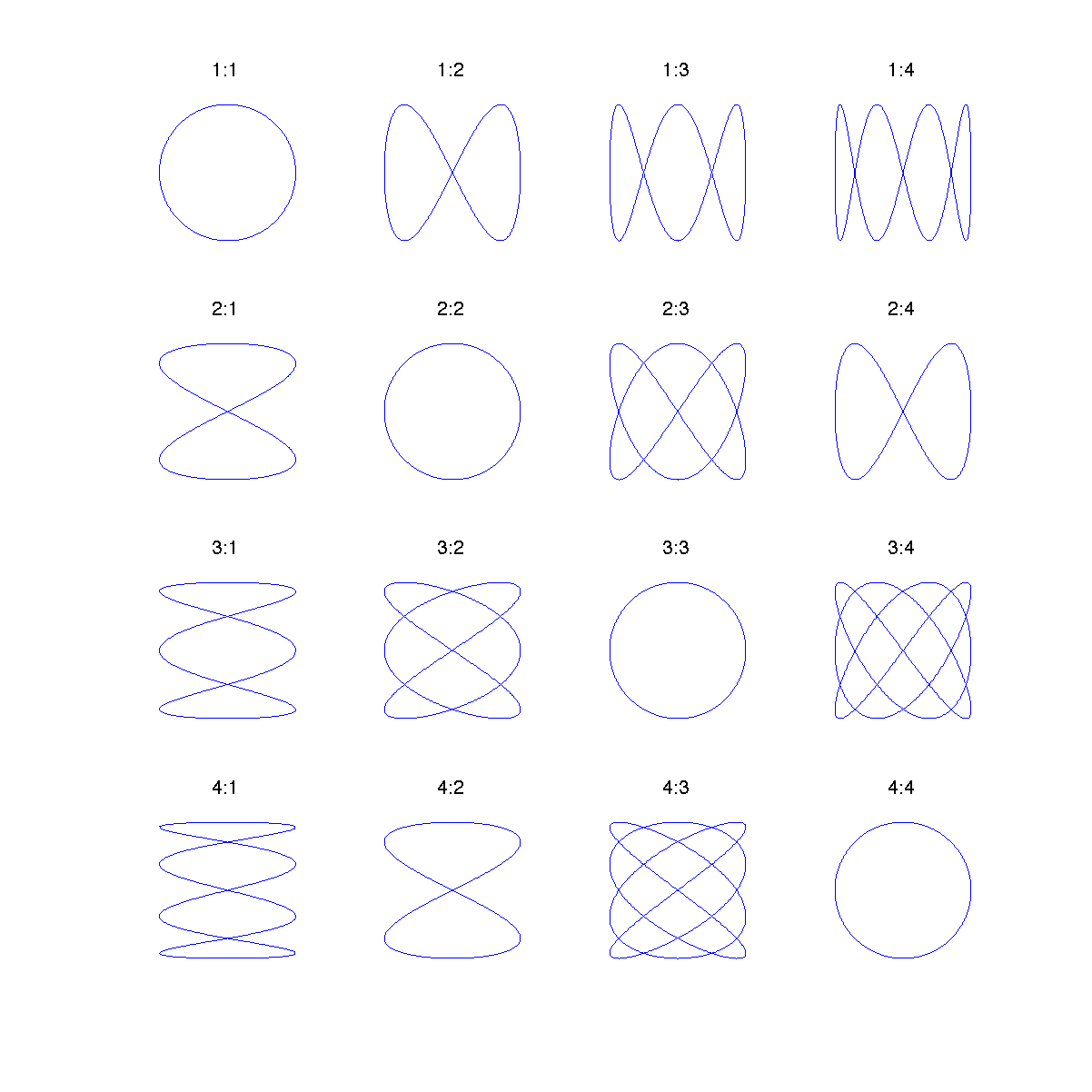# plot10.m - Plot a Grid of Lissajous FiguresThe code:
```N = 4;            % Number of plots along x axis
M = 4;            % Number of plots along y axis

for kx = 1:N
for ky = 1:M
% The period of the pattern will be 2*pi, unless the two wavenumbers
% share a common divisor. If they do share a common divisor, then the
% period will be reduced by that common factor. Use the greatest
% common divisor function gcd().
period = 2*pi/gcd(kx,ky);
t = linspace(0,period,1000);

% figure out the phase shift that "rounds out" the pattern
phase = ky*period/4;
x = sin(kx*t);
y = sin(ky*t + phase);

% plot
subplot(N, M, (kx-1)*M+ky)
plot(x,y,'-')
axis equal
axis([-1.2 1.2 -1.2 1.2])
title([num2str(kx) ':' num2str(ky)])

% turn off the axes
axis off
end
end
```

This program extends the example plot09.m to show a grid of Lissajous figures all on the same plot. The number of plots shown may be controlled by changing N and M (try it). The command axis off is used to turn off the plot axes making the plot look a little cleaner and easier to read.

Monkey Home   |    Prev   |   Next